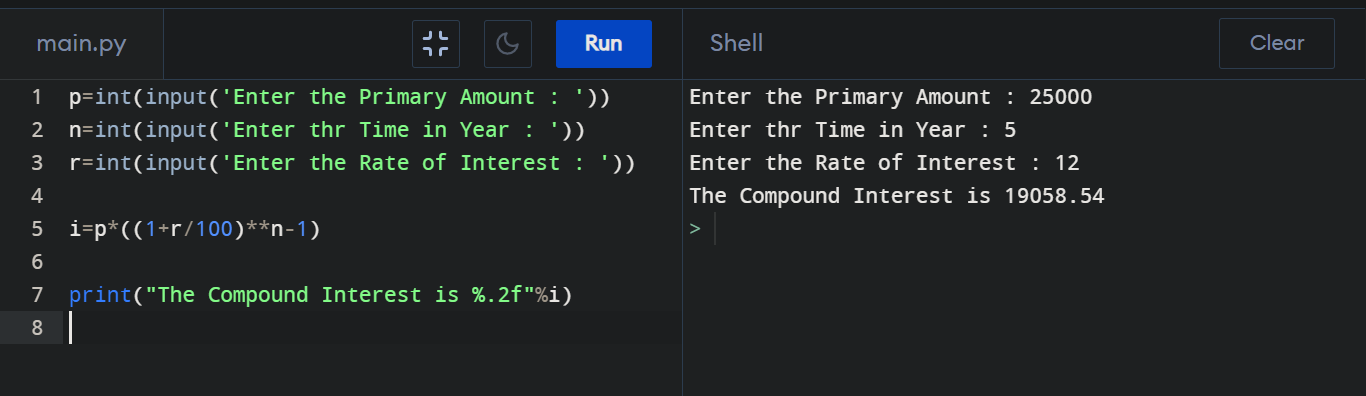Python Program to Calculate Compound Interest (CI)

How can we find the Compound Interest? We can do it by applying the following formula, Compound Interest = Principal Amount * ( 1 + Rate of Interest )Number of years )

Compound Interest using Python :

p=int(input("Enter the Primary Amount : "))
n=int(input("Enter thr Time in Year : "))
r=int(input("Enter the Rate of Interest : "))

i=p*((1+r/100)**n-1)

print("The Compound Interest is %.2f"%i)

Output :